# EQULIBRIUM

Moment of a Force

The Effects of Turning Forces
Explain the effects of turning forces
force is a push or pull which when applied to a body it will change its state either by stopping it if it was in motion or making it move if it was at rest.
If a body under the action of a net externalforceis allowed to rotate about a pivot, the body will tend to turn in the direction of the applied force.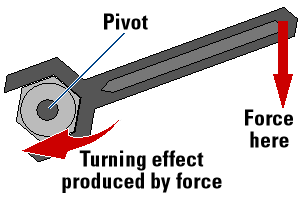Examples of turning effect of force:
• A person pushing a swing will make the swing rotate about its pivot.
• A worker applies force to a spanner to rotate a nut.
• A person removes a bottle’s cork by pushing down the bottle opener’s lever.
• Force is applied to a door knob and the door swings open about its hinge.
• A driver can turn a steering wheel by applying force on its rim.

Moment of a force
The moment of a force about a point is given by the product of the force's magnitude and the perpendicular distance between the line of action of the force and the point.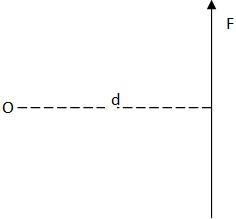The perpendicular distance between the line of action of the force 'F' and the point 'O' is 'd'.
The moment of the force is therefore = force x Distance.
Moment of force = Force x Distance (N X m)
The SI unit of moment of a force is Nm
Example

1. The line of action of a force 48N is at perpendicular distance of 1.5m from the point. Find the moment of the force about the point.
Data given
Magnitude of the force = 48N
Perpendicular distance = 1.5m.
Find moment of a force =?
:Moment of a force = force x Distance
= 48 N x 1.5 M

Moment of the force = 72Nm

2. The moment of a force about a point is 1120Nm. If the magnitude of a force is 5600N, find the perpendicular distance between the point and the line of action of the force.
Data given
Moment of a force = 1120Nm
Magnitude of a force = 5600N
Perpendicular distance =?
From: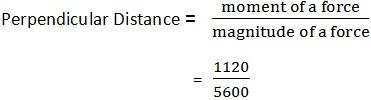Perpendicular distance = 0.2m
3. The moment of a force is 1000 Nm. If the line of the force is at perpendicular distance of 100m, find the magnitude of a force.
Data given
Moment of a force =1000Nm
Perpendicular distance =100m
Magnitude of a force =?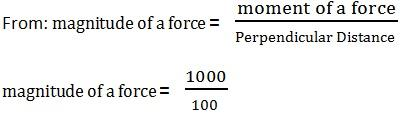Magnitude of a force is 10N.
The principal of Moments
The Principle of moments states that:”If a body is in equilibrium under a force which lie in one plane, the sum of the clockwise moments is equal to the sun of the anticlockwise moments about any point in that plane.”
Activity 1
Experiment.
Aim: To determine the moment of a force.
Materials and apparatus:Meter rule, several different weights, inelastic cotton thread, knife edge and a marker pen.
Procedures
• Balance the meter rule horizontally on a knife edge.
• Mark a balance point as C. Use the marker pen to do that.
• Suspend a meter rule from a fixed axis through C. Suspend unequal weights W₁ and W₂ from the meter rule by using thin cotton threads.
• Adjust the distance d₁ and d₂ of the weights W₁ and W₂ from C until the meter rule balance.
• Repeat the experiment five times using different values of W₁ and W₂.Record the results on the table as shown below.
 W₁(g) W₂(g) d₁(cm) d₂(cm) W₁ d₁ (gcm) W₂ d₂ (gcm)
Observation:In each case it will be found that W₁ d₁ is equal to W₂ d₂.

The Principle of Moment in Daily Life
Apply the principle of moment in daily life
Moment of a force is used in the following:
• Is applied by a hand to unscrew a stopper on the bottle.
• Is applied by a spanner to unscrew a nut on a bottle.
• Turning a steering wheel of a car.
The principle of moments states that “ If a body is in equilibrium under the action of forces which lie in one plane, the sum of the clockwise moments is equal to the sum of the anti clockwise moments about any point in that plane".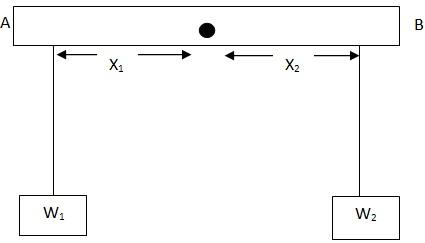M is the point of fulcrums.
Clockwise moment = W2 x X2
Anti clockwise moment = W1 x X1
Sum of clockwise Moment = Sum of anticlockwise Moment
W2 x X2 = W1 x X1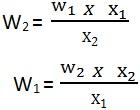Examples:
1. 100g weight is suspended 45 cm from the pivot A of a light rot. If a weight is suspended 20.5cm from the point balance the 100g weight, determine the W1 if a 300g weight is used to balance the 100g weight, determine the distance of the 300g weight from the point.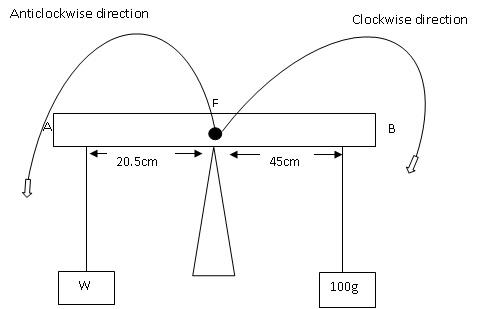Clock wise moment   = 100g x 45cm
Anticlockwise moment =   w x 20.5cm
Sum of clockwise Moment = anticlockwise moment
100s x 45cm =   20.5Wcm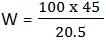W = 220g

2. If a 100g weight is used to balance the weight determine the distance of the 300g weight from the point.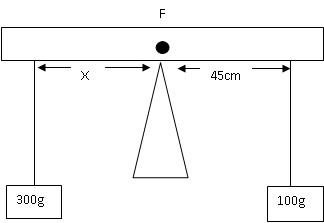Clock wise moment = anticlockwise moment
100g x 45cm = 300g x X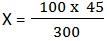The distance from the pivot is 15cm
EXAMPLE
Anticlockwise   = 15cm and 300g
Clockwise = 100g and 45cm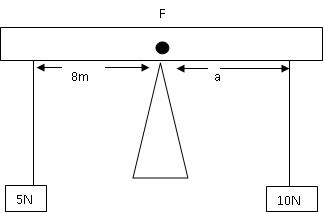Solution:
Clockwise moment = ant clockwise moment
a x 10N = 8M x 5N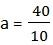a = 4m
The distance required was 4m
UNIFORM BEAM
Example
1. A heavy uniform metal beam AB weighting 500kg if is supported at it ends. The beam carries a weight of 3000kg at distance of 1.5m from the end of A. If the beam is 4m long, determine the thrust on the supports A and B.Since the beam is uniform its weight acts vertically down ward at the middle.
-let the thrust at point A be 2 and the point B be Y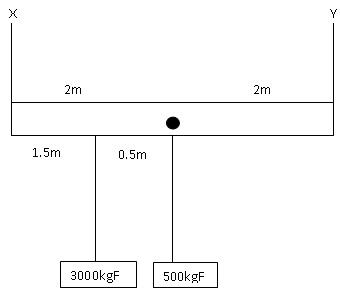Taking moment about point A
Clock wise moment 3000kg x 1.5m + 500kg x 2m
Anti clock wise = Y x 4M
Clock wise moment = Anti clock wise moment
3000kgF x 1.5m + 500kgF x 2 = Y x 4m
4500 + 1000=Y4m
5500kgm = Y4m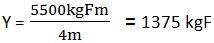Down force = upward force
(3000 + 500) =X + 1375
3500  = X + 1375
X = 3500 -1375
Then the force required is = 2125kgF
2. A uniform half – meter, rule AB is balanced horizontally on a knife edge placed 5cm from B with a mass of 80g at B.find the mass of the ruler.Clockwise moment = Anticlockwise moment

80g x 5cm = m x 20
400cm = 20 x M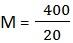M = 20g
Exercise

1. A uniform bar AB of height 5m weights 60N. The bar is supported at a horizontal position by two vertical strings X and Y. If string X is 0.6m from A and string Y is 1.8m from B. Find the tension in the string.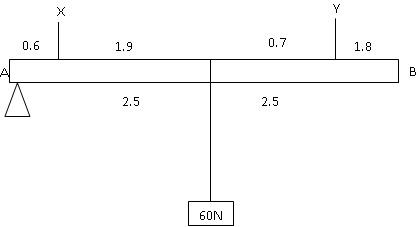Moment about A
Clock wise Moment = 60 x 2.5
Anti clockwise Moment = 0.6 x X + 3.2Y
Clockwise moment = anticlockwise moment
60 x 2.5 = 0.6 x X + 3.2y………(1)
X + Y =60…………(2)
X = 60 – Y
150 =0.6X + 3.2Y     substitute the value of X to the equation 2
150 = 0.6(0.6 – Y) +3. 2Y
150 =36 – 0.6Y +3.2Y
150 =36 + 2.6Y
114 = 2.6Y
Y = 43.84 then
= 60 -48.84
= 16.15
Tensions is the string = 16.5N

COUPLES
A couple consists of two equal and opposite parallel forces and it has turning effect.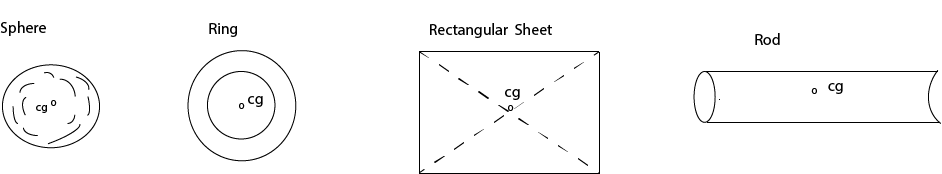Centre of gravity of regular objects.

Irregular shaped bodies.
Locating centre of gravity of a cardboard.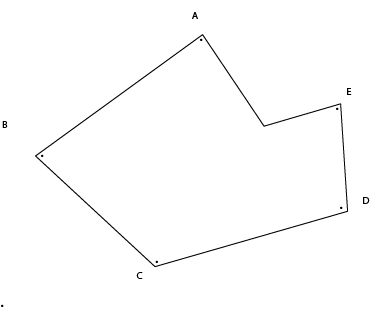Steps
1. Punch holes on the corner points ABCDE.
2. Run a pencil through the first hole (A) and let the cardboard hang freely.
3. Attach a small mass to one end of a string and suspend it by tying the other end on that pencil.
4. Using a felt pen draw a line on the cardboard along the string.
5. Repeat the procedure 2 - 4 above using the holes B, C, D and E.
The point where the lines will meet is the centre of gravity of a cardboard.

Conditions for equilibrium

First Condition
The sum of forces acting the object is zero.

Second Condition
The sum of the moment acting on an object is zero.